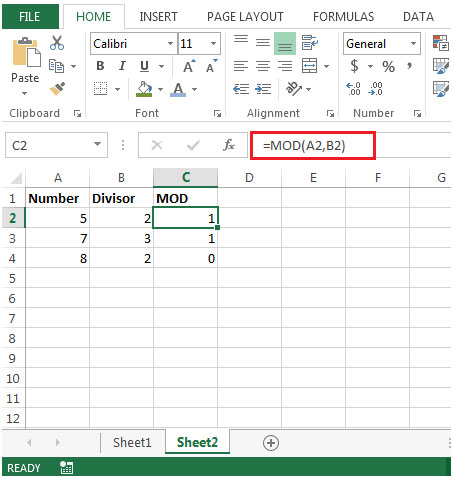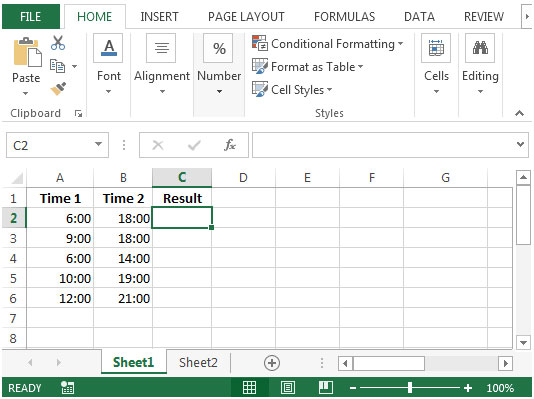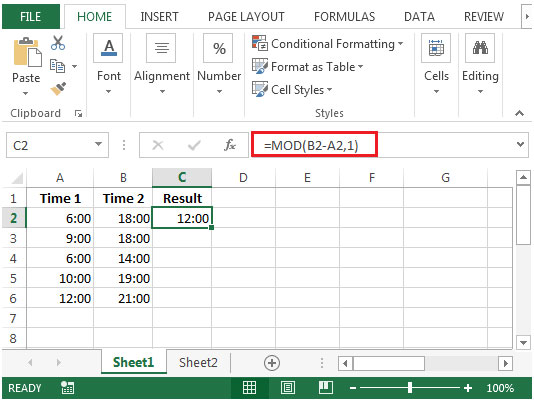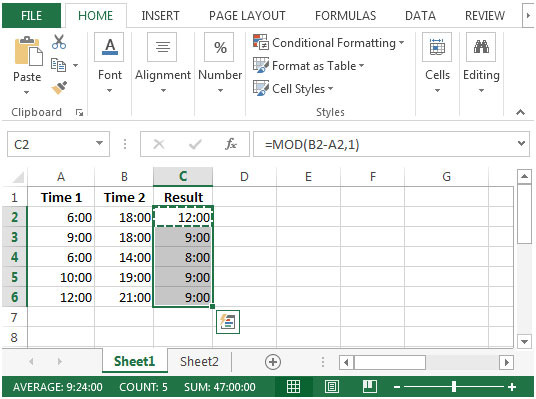# Avoiding Errors when Subtracting Times in Microsoft Excel

In this article, we will learn how to avoid errors when subtracting times in Microsoft Excel.

While calculating the difference between two time values, the former time must be subtracted from the latter one to avoid any error.

However, when the latter time refers to the following day (with respect to the earlier time), an error will occur.

We want to create a formula that avoids these errors when calculating the difference between any two time values.
We will use MOD function to get the output.

MOD: Returns the remainder after a number is divided by a divisor.

Syntax =MOD(number,divisor)

number: It is a numeric value whose remainder you want to find.

divisor: It is the number which is used to divide the number argument.

Example of Mod Function:Let us take an example:

• We have time in column A & B
• We want to find their difference• We will enter the formula in cell C2=MOD(B2-A2,1)• Copying the formula down in below range of cells, we will get the desired result1.Great!

2.This method does not achieve the desired result.

You begin by stating that that an earlier (former) time must be subtracted from a later (latter) time to avoid an error.
In other words, 6:00 - 3:00 = 3:00. But 3:00 - 6:00 cannot equal -3:00 because there are no negative times, therefore Excel generates an error.

The problem, then, that this tip seeks to solve, is to find the difference between 6:00 today and 3:00 tomorrow because, as noted, ignoring the dates, 3:00-6:00 will generate an error.

You propose to solve this problem using the MOD function, which returns the Remainder of an operation where a number is divided by a divisor.

You give examples such as 5/2=2 1/2. Or, from the MOD function, the result would be 1, the Remainder of the division operation.

So far, so good.

But then you set up your time calculation table, with earlier (former) times in column A, and later (latter) times in column B. You needlessly use the MOD function because the formula B2-A2 is subtracting the earlier (former) from the later (latter). Your numbers with that formula would never generate an error to begin with.

If, in cell C2, you were to input the formula =B2-A2, then copy that formula on down through C6, you would get exactly the same results that you show in your final screenshot.

Your use of the MOD function only divides the difference between B2 and A2 by 1. That =MOD(B2-A2,1) gives you a result instead of an error is strange. 18:00-6:00=12:00. 12:00/1=12:00 with no remainder. It appears that when dividing by 1, Excel treats the entire Numerator as a Remainder, which is incorrect.

Further, the problem you're trying to solve is subtracting a time of the following day from a time today when those times would otherwise generate an error. 6:00-18:00 yields a negative. Unless 18:00 is tomorrow's time. The actual length of time between 6:00 today and 18:00 tomorrow is 36 hours.

Which raises the question of what you actually wanted to achieve here. Rather than determing the actual difference between two times on different dates, it looks like what you're after is the Absolute Value of the difference between two times regardless of their date. Which would make the ABS function a more apt tool for the problem.

3.WHY MY RESULT IS #VALUE?

Terms and Conditions of use

The applications/code on this site are distributed as is and without warranties or liability. In no event shall the owner of the copyrights, or the authors of the applications/code be liable for any loss of profit, any problems or any damage resulting from the use or evaluation of the applications/code.